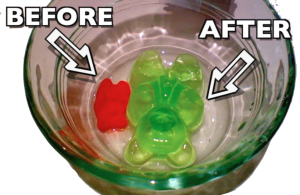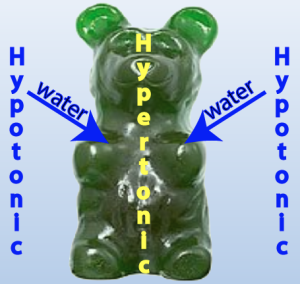## 1. A gummy bear mystery

If you take a gummy bear, and soak it in water for a few hours, a remarkable change will occur. As you can see, the gummy bear in water will increase in size. If you weighed it, you’d find that its mass increases up to five times. What’s going on?The red gummy bear hasn’t been soaking in water. The green gummy bear has been soaking in water for several hours.

## 2. Osmosis is water flow from hypotonic to hypertonic

Through osmosis, water flows from higher to lower concentration.

Consider the diagram to the left. It’s a side view of a beaker that holds two solutions. The solutions are separated by a selectively permeable membrane. The membrane allows water to pass through, but not the solute, which is represented by the red spheres.

As you can see, there’s more solute on side 1 than side 2. If there are more solute molecules, then there have to be less water molecules. Here’s a summary of the situation:

 Side 1 Side 2 Higher percentage of solute molecules Lower percentage of solute molecules Lower percentage of water molecules Higher percentage of water molecules

A somewhat outdated way to refer to a solute is as the tonic (a phrase we hold onto in phrases like “gin and tonic,” where the tonic is dissolved in the gin). Using that, we wind up with two more descriptions of the situation above:

• Side 1 is hypertonic to side 2. That means that the solution on side 1 has more solute dissolved in it than the solution on side 2.
• Side 2 is hypotonic to side 1. That means that the solution on side 2 has less solute dissolved in it than the solution on side 1.

You can remember this by focusing on the prefixes hypo and hyper.

• Hypo means under, or beneath, as in hypodermic (beneath the skin). Hypotonic means less solute.
• Hyper means too much, as in hyperactive (too active). Hypertonic means more solute.Because the gummy bear is hypertonic to the surrounding water, water will flow into the gummy bear.

Since water (like everything else) diffuses from higher concentration to lower concentration, we can expect that water will flow from side 2 to side 1. Another way to say that is that water will diffuse from the hypotonic side to the hypertonic side.

That’s why the green gummy bear expanded. Water flowed from the water in the cup into the gummy bear. Why? Because the water in the cup was hypotonic to the gummy bear (which was hypertonic to the water in the cup). To accommodate this inflow of water, the gummy expanded.

The solutions on opposite sides of a membrane can also have the same concentration of water, which is to say that they can have the same concentration of dissolved solute. The word for that is isotonic. Here’s a diagram of two solutions that are isotonic to one another.

SUMMARY:

1. Osmosis is the diffusion of water.
2. Hypertonic solutions have relatively more dissolved solute than an adjacent solution.
3. Hypotonic solutions have relatively less dissolved solute than an adjacent solution
4. Isotonic solutions have equal amounts of dissolved solutes
5. Water always diffuses from hypotonic to hypertonic.

## 3. Osmosis Vocabulary Quiz

This quiz will help you master the following terms. Hover your mouse over any term below to see a definition.

1. Diffusion
2. Osmosis
3. Solvent
4. Solute
5. Solution
6. Hypertonic
7. Hypotonic
8. Isotonic

About half of the questions ask you to describe or define a term. Write out your answer, and then check it against mine. If you understand the concept, click “Got it.” Otherwise, click “need more practice.

## Osmosis Vocabulary Quiz

[qwiz random = “true” qrecord_id=”sciencemusicvideosMeister1961-Osmosis Vocabulary”]

[h]Osmosis Vocabulary

[i]

[!] Card 1+++++++++++++++++++++++++++++++++++[/!]

[q]The movement of a substance from higher concentration to lower concentration is ________________

[hangman]

[c]diffusion

[!] Card 2+++++++++++++++++++++++++++++++++++[/!]

[q]The diffusion of water is

[hangman]

[c]osmosis

[!] Card 3+++++++++++++++++++++[/!]

[q]A mixture in which one thing is dissolved in another is a(n) ________________

[hangman]

[c]solution

[!] Card 4+++++++++++++++++++++[/!]

[q]In a solution, the thing that does the dissolving is the

[hangman]

[c]solvent

[!] Card 5+++++++++++++++++++++[/!]

[q]In a solution, the thing that gets dissolved is the

[hangman]

[c]solute

[!] Card 6+++++++++++++++++++++[/!]

[q]In lemonade (that you make from a mix), the lemonade mix is the _____________.

[hangman]

[c]solute

[!] Card 7+++++++++++++++++++++[/!]

[q]In lemonade (that you make from a mix), the water is the _____________.

[hangman]

[c]solvent

[!] Card 8+++++++++++++++++++++[/!]

[q]Solution “A” has more dissolved solute than solution “B.” Solution “A” is __________ to solution “B.”

[hangman]

[c]hypertonic

[!] Card 9+++++++++++++++++++++[/!]

[q]Solution “A” has less dissolved solute than solution “B.” Solution “A” is __________ to solution “B.”

[hangman]

[c]hypotonic

[!] Card 10+++++++++++++++++++++[/!]

[q]Solution “A” has the same amount of dissolved solute as solution “B.” Solution “A” is __________ to solution “B.”

[hangman]

[c]isotonic

[!] Card 11+++++++++++++++++++++[/!]

[q]Solution “A” has a higher percentage of water than solution “B.” Solution “A” is __________ to solution “B.”

[hangman]

[c]hypotonic

[!] Card 12+++++++++++++++++++++[/!]

[q]Solution “A” has a lower percentage of water than solution “B.” Solution “A” is __________ to solution “B.”

[hangman]

[c]hypertonic

[!] Card 22+++++++++++++++++++++[/!]

[q]If I want to make a solution more hypertonic, I add more _____________.

[hangman]

[c]solute

[!] Card 23+++++++++++++++++++++[/!]

[q]If I want to make a solution more hypotonic, I add more _____________.

[hangman]

[c]solvent

[!] Card 24+++++++++++++++++++++[/!]

[q]If I want to make a solution less hypertonic, I add more _____________.

[hangman]

[c]solvent

[!] Card 12+++++++++++++++++++++[/!]

[q]Define diffusion. Use the word “concentration” in your definition.

[f]Diffusion is the movement of a substance from higher to lower concentration.

[!] Card 13+++++++++++++++++++++[/!]

[f]Diffusion is the movement of a substance down its concentration gradient.

[!] Card 14+++++++++++++++++++++[/!]

[q]Define osmosis.

[f]Osmosis is the diffusion of water.

[!] Card 15++++++++hypotonic+++++++++++++[/!]

[q]Using two imaginary solutions, “A” and “B,” define hypotonic in terms of dissolved solute.

[f]If solution “A” has less dissolved solute than solution “B,” then solution “A” is hypotonic to solution “B”

[!] Card 16+++++++++hypotonic++++++++++++[/!]

[q]Using two imaginary solutions, “A” and “B,” define hypotonic in terms of water concentration.

[f]If solution “A” has a higher water concentration than solution “B,” then solution “A” is hypotonic to solution “B.”

[!] Card 17+++++++hypertonic++++++++++++++[/!]

[q]Using two imaginary solutions, “A” and “B,” define hypertonic in terms of dissolved solute.

[f]If solution “A” has more dissolved solute than solution “B,” then solution “A” is hypertonic to solution “B.”

[!] Card 18+++++++hypertonic++++++++++++++[/!]

[q]Using two imaginary solutions, “A” and “B,” define hypertonic in terms of water concentration.

[f]If solution “A” has a lower water concentration than solution “B,” then solution “A” is hypertonic to solution “B.”

[!] Card 19+++++++isotonic++++++++++++++[/!]

[q]Using two imaginary solutions, “A” and “B,” define isotonic in terms of dissolved solute.

[f]If solution “A” has the same amount of dissolved solute as solution “B,” then solution “A” is isotonic to solution “B.”

[!] Card 20+++++++isotonic++++++++++++++[/!]

[q]Using two imaginary solutions, “A” and “B,” define isotonic in terms of water concentration.

[f]If solution “A” has the same water concentration as solution “B,” then solution “A” is isotonic to solution “B.”

[!] Card 21+++++++++++++++++++++[/!]

[q]Using the terms hypotonic and hypertonic, describe how water flows.

[f]Water always flows from hypotonic to hypertonic.

[x]